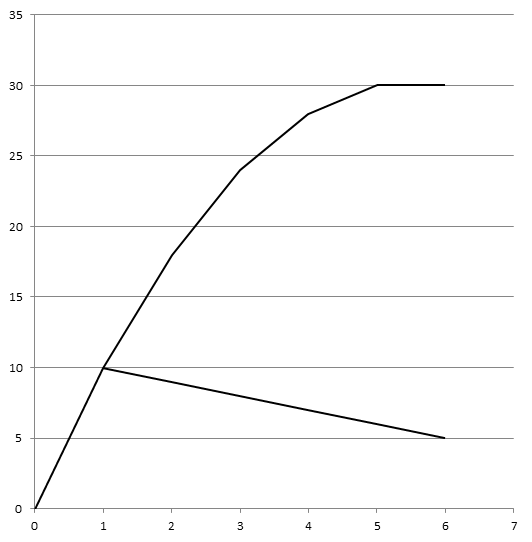# Concept of short-run total cost curves

Concept of short-run total cost curves:

1. Total fixed cost (TFC):
It refers to the total expenditure made by a firm or industry to purchase the fixed factors of production for the production of commodity. In short run, it remain constant whatever change in output.
We know,
TC = TFC + TVC
TFC = TC – TVC

2. Total variable cost (TVC):
It refers to the total expenditure made by a firm or industry increased on the variable factor of production for production of commodity. In short-rum it is variable whatever change in output.

It remains zero if there is no production.

We know,
TC = TFC + TVC
TVC = TC – TFC

3. Total cost (TC):
It refers to the total expenditure made by firm to purchase the all kinds of variable and fixed factors of production for the production of commodities. In other word it is the summation of TFC and TVC.

We can explain the concept of cost and can derive total cost curve by the help of given table:

 Output Production TFC TVC TC = TFC + TVC 0 70 0 70 1 70 30 100 2 70 40 110 3 70 45 115 4 70 55 125 5 70 75 145 6 70 125 195

Note: All the amounts are in lakhs.

In the above table, TFC is same at every level of output. TVC is zero at zero level of production and rises as output rises. TC is equal to TFC and TVC. On the basis of above table we can derive the total cost curves.

We can describe with the help of given figure:On the above figure, output and cost are measured on x and y-axis respectively. TFC curve is parallel to x-axis indicating that it remains constant at any quantity of output produced.

TVC curve increases S-shaped, due to operation of law of variable proportion in production. TVC starts from origin which shows TVC is zero when output is zero.

TC curve is derived by adding the TFC and TVC curve. Since, TFC remains constant even if output is zero. TC curve has same shape as that of TVC.